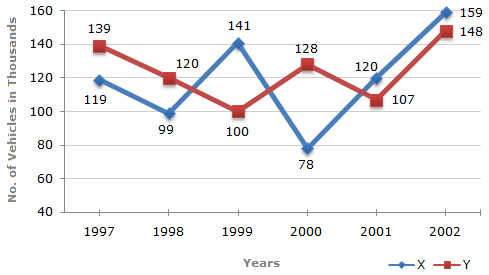# Data Interpretation - Line Charts - Discussion

### Discussion :: Line Charts - Line Chart 3 (Q.No.2)

Study the following line graph and answer the questions based on it.

Number of Vehicles Manufactured by Two companies ove the Years (Number in Thousands)2.

What is the difference between the total productions of the two Companies in the given years ?

 [A]. 19000 [B]. 22000 [C]. 26000 [D]. 28000

Answer: Option C

Explanation:

From the line-graph it is clear that the productions of Company X in the years 1997, 1998, 1999, 2000, 2001 and 2002 are 119000, 99000, 141000, 78000, 120000 and 159000 and those of Company Y are 139000, 120000,100000, 128000, 107000 and 148000 respectively.

Total production of Company X from 1997 to 2002

= 119000 + 99000 + 141000 + 78000 + 120000 + 159000

= 716000.

and total production of Company Y from 1997 to 2002

= 139000 + 120000 + 100000 + 128000 + 107000 + 148000

= 742000.

Difference = (742000 - 716000) = 26000.

 Zakaria said: (Apr 29, 2012) You can calculate this way- difference of each year= 20+21-41+50-13-11=26 Ans= 26 thousand.

 Prachi Thote said: (Jun 25, 2020) 119000 + 99000 + 141000 + 78000 + 120000 + 159000 = 796000. So the difference is 54000.

#### Post your comments here:

Name *:

Email   : (optional)

» Your comments will be displayed only after manual approval.

#### Current Affairs 2021

Interview Questions and Answers